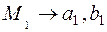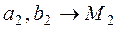CATEGORIES:

LECTURE 3.

The simplest problem of analytic geometry. Equations of a straight line on a plane.

LECTURE PLAN:

1. The simplest problem of analytic geometry

2. Equations of a straight line on a plane

Analytic Geometry in the Plane

Consider the Cartesian rectangular coordinate system in the plane. Taking the projection of any point М1 on the x and y coordinate we obtain two numbers x=a1 and y=b1. Take two numbers a2 and b2 plot a2 on the x-axis and b2 on the y-axis . Having drawn two straight lines parallel to the coordinate axes through these points we find obtain a point M2 in their intersection.

 a2
 M2(a2;b2)b2
 M1(a1;b1) b1x

0

 a1Thus, there is a one–to–one correspondence between points in the plane and pairs of numbers.

Date: 2015-01-02; view: 877

 <== previous page | next page ==> Inverse Matrices | The distance between two points. Let us find the distance between two points М1 and М2 in the plane.
doclecture.net - lectures - 2014-2022 year. Copyright infringement or personal data (0.002 sec.)